# Frank solutions for ICSE Class 10 Mathematics Part 2 chapter 17 - Circles [Latest edition]

#### Chapters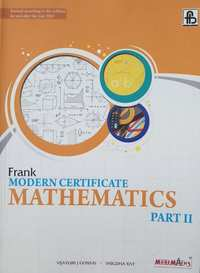## Chapter 17: Circles

Exercise 17.1Exercise 17.2Exercise 17.3
Exercise 17.1

### Frank solutions for ICSE Class 10 Mathematics Part 2 Chapter 17 Circles Exercise 17.1

Exercise 17.1 | Q 1.1

Find the length of the chord of a circle in the following when:

Radius is 13 cm and the distance from the centre is 12 cm

Exercise 17.1 | Q 1.2

Find the length of the chord of a circle in the following when:

Radius is 1. 7cm and the distance from the centre is 1.5 cm

Exercise 17.1 | Q 1.3

Find the length of the chord of a circle in the following when:

Radius is 6.5 cm and the distance from the centre is 2.5 cm

Exercise 17.1 | Q 2

AB and CD are two equal chords of a drde intersecting at Pas shown in fig. P is joined to O , the centre of the cirde. Prove that OP bisects  ∠ CPB.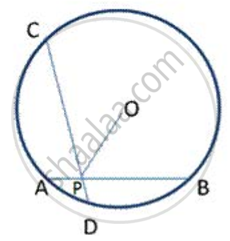Exercise 17.1 | Q 3

In fig. the centre of the circle is O. PQ and RS are two equal chords of the circle which , when produced , meet at T outside the circle . Prove that (a) TP = TR (b) TQ = TS.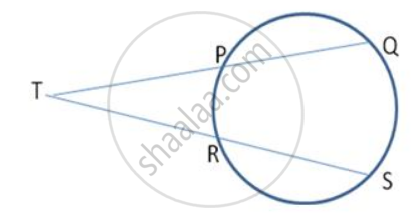Exercise 17.1 | Q 4

PQ and QR are two equal chords of a circle. A diameter of the circle is drawn through Q . Prove that the diameter bisects ∠ PQR.

Exercise 17.1 | Q 5

M and N are the midpoints of chords AB and CD . The line MN passes through the centre O . Prove that AB || CD.

Exercise 17.1 | Q 6

Prove that the line segment joining the midpoints of two parallel chords of a circle passes through its centre.

Exercise 17.1 | Q 7

Two congruent drdes have their centres at 0 and P. Mis the midpoint of the line segment OP. A straight line is drawn through M cutting the two circles at the points A, B, C and D. Prove that the chords AB and CD are equal.

Exercise 17.1 | Q 8

Two circles with centres O and P intersect each other at A and B as shown in following fig. Two straight lines MAN and RBQ are drawn parallel to OP.
Prove that (i) MN = 20 P (ii) MN= RQ.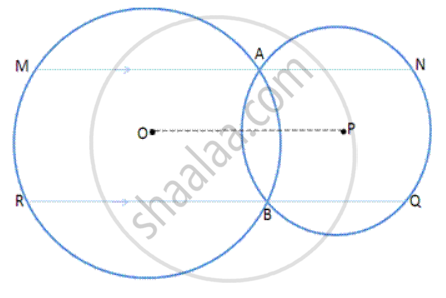Exercise 17.1 | Q 9

In following fig. ABC is an equilateral triangle . A circle is drawn with centre A so that ot cuts AB and AC at M and N respectively. Prove that BN = CM.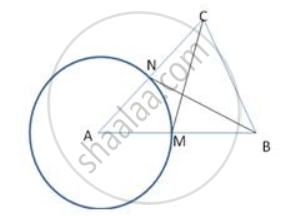Exercise 17.1 | Q 10

In following fig., ABCD is a square. A cirde is drawn with centre A so that it cuts AB and AD at Mand N respectively. Prove that  Δ DAM  ≅  Δ .BAN.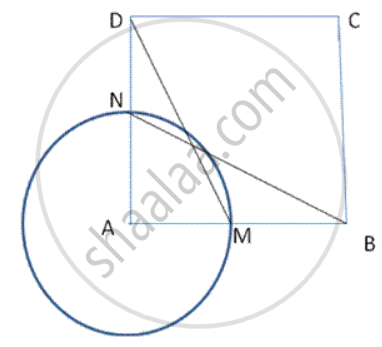Exercise 17.1 | Q 11

In fig, AB and CD are two equal chords of a circle with centre O. If M and N are the midpoints of AB and CD respectively,

prove that (a) ∠ ONM = ∠ ONM (b) ∠ AMN = ∠ CNM.

Exercise 17.1 | Q 12

Find the diameter of the circle if the length of a chord is 3.2 cm and itd distance from the centre is 1.2 cm.

Exercise 17.1 | Q 13

In following figure .,XY and YZ are two equal chords of a circle with centre O. Prove that the bisector of ∠ XYZ passes through O.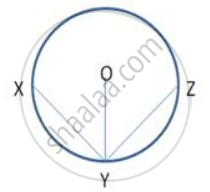Exercise 17.1 | Q 14

In figure , ABC is an isosceles triangle inscribed in a circle with centre O such that AB = AC = 13 cm and BC = 10 cm .Find the radius of the circle.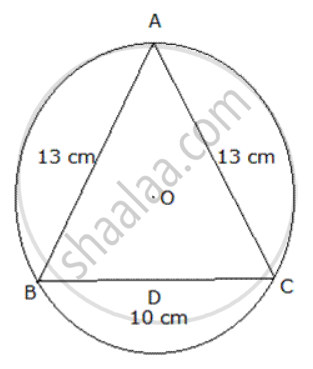Exercise 17.1 | Q 15

AB and AC are two equal chords of a circle with centre o such that LABO and LCBO are equal. Prove that AB = BC.

Exercise 17.1 | Q 16

A chord of a length 16.8 cm is at a distance of 11.2 cm from the centre of a circle . Find the length of the chord of the same circle which is at a distance of 8.4 cm from the centre.

Exercise 17.1 | Q 17

A chord of length 6 cm is at a distance of 7.2 cm from the centre of a circle. Another chord of the same circle is of length 14.4 cm. Find its distance from the centre.

Exercise 17.1 | Q 18

A chord of length 8cm is drawn inside a circle of radius 6cm. Find the perpendicular distance of the chord from the centre of the circle.

Exercise 17.1 | Q 19

Two circles of radii 5cm and 3cm with centres O and P touch each other internally. If the perpendicular bisector of the line segment OP meets the circumference of the larger circle at A and B, find the length of AB.

Exercise 17.1 | Q 20

Two chords AB and CD of lengths 6cm and 12cm are drawn parallel inside the circle. If the distance between the chords of the circle is 3cm, find the radius of the circle.

Exercise 17.1 | Q 21

Two chords of lengths 10cm and 24cm are drawn parallel o each other in a circle. If they are on the same side of the centre and the distance between them is 17cm, find the radius of the circle.

Exercise 17.1 | Q 22

In following figure , AB , a chord of the circle is of length 18 cm. It is perpendicularly bisected at M by PQ.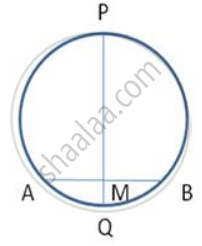Exercise 17.2

### Frank solutions for ICSE Class 10 Mathematics Part 2 Chapter 17 Circles Exercise 17.2

Exercise 17.2 | Q 1

In following figure , O is the centre of the circle. If ∠ APB = 50° then find ∠ AOB and ∠ OAB.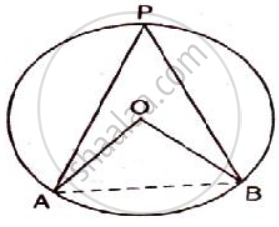Exercise 17.2 | Q 2

In a cyclic quadrilateral ABCD , AB || CD and ∠ B = 65 ° , find the remaining angles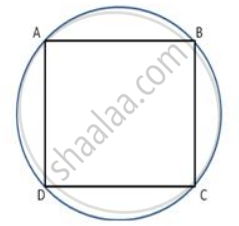Exercise 17.2 | Q 3

In a cyclic quadrilateral ABCD , AB || CD and ∠ B = 65° , find the remaining angles.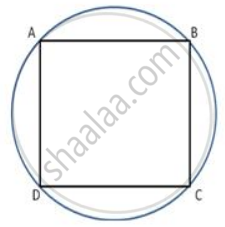Exercise 17.2 | Q 4

In a cyclic quadrialteral ABCD , if m ∠ A = 3 (m ∠C). Find m ∠ A.

Exercise 17.2 | Q 5

In a circle with centre O , chords AB and CD intersets inside the circle at E . Prove that ∠ AOC = ∠ BOD = 2 ∠ AEC.

Exercise 17.2 | Q 6

In the given Figure, AB and CD are two chords of a circle, intersecting each other at P such that AP = CP. Show that AB= CD.

Exercise 17.2 | Q 7

MABN are points on a drde having centre O. AN and MB cut at Y. If ∠ NYB = 50" and ∠ YNB = 200, find ∠  MAN and reflex angle MON.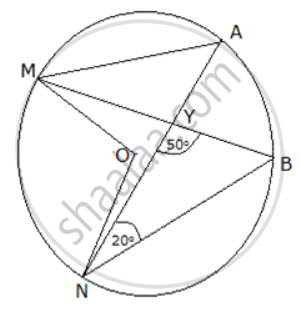Exercise 17.2 | Q 8

In fig., PT is a tangent to the circle at T and PAB is a secant to the same circle. If PB = 9cm and AB = 5 cm, find PT.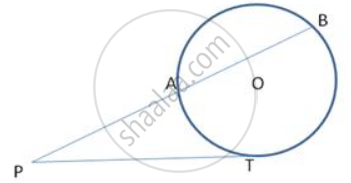Exercise 17.2 | Q 9

Two circles are drawn with sides AB, AC of a triangle ABC as diameters. They intersect at a point D. Prove that D lies on BC.

Exercise 17.2 | Q 10

Prove that the circle drawn on any one of the equal sides of an isosceles triangle as diameter bisects the base.

Exercise 17.2 | Q 11

Prove that the circle drawn with any side of a rhombus as a diameter, passes through the point of intersection of its diagonals.

Exercise 17.2 | Q 12

In fig., O is the centre of the circle and ∠ AOC = 1500. Find ∠ ABC.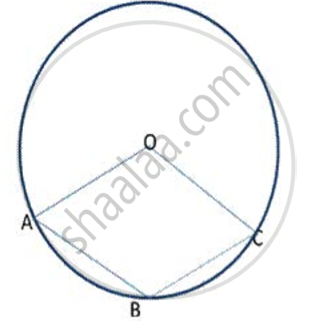Exercise 17.2 | Q 14

In triangle ABC, AB = AC. A circle passing through B and c intersects the sides AB and AC at D and E respectively. Prove that DE || BC.

Exercise 17.2 | Q 15

The bisectors of the opposite angles A and C of a cydic quadrilateral ABCD intersect the cirde at the points E and F, respectively. Prove that EF is a diameter of the circle.

Exercise 17.2 | Q 16

Prove that the angles bisectors of the angles formed by producing opposite sides of a cyclic quadrilateral (provided they are not parallel) intersect at right triangle.

Exercise 17.2 | Q 17

Bisectors of angles A, B and C of a triangle ABC intersect its circumcircle at D, E and F respectively. Prove that the angles of  Δ DEF are 90° - "A"/2 , 90° - "B"/2 and 90° - "C"/2 respectively.

Exercise 17.2 | Q 18

In following figure . O is the centre of the circle. Find ∠ BAC.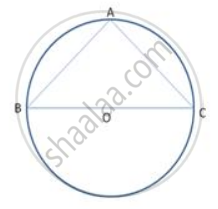Exercise 17.2 | Q 19

In following  fig., O is the centre of the circle, prove that ∠x =∠ y + ∠ z.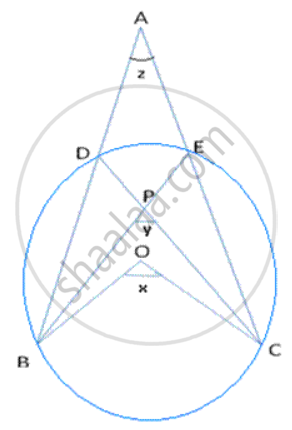Exercise 17.2 | Q 20

In following figure , chord ED is parallel to the diameter AC of the circle. Given ∠ CBE = 65° , calculate ∠DEC .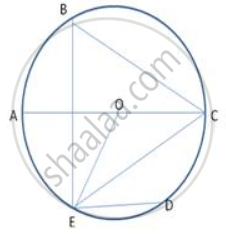Exercise 17.2 | Q 21

In following figure , C is a point on the minor arc AB of the circle with centre O . Given ∠ ACB = p° , ∠  AOB = q° , express q in terms of p. Calculate p if OACB is a parallelogram.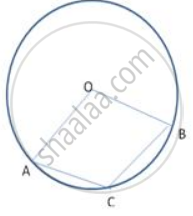Exercise 17.2 | Q 22

In following figure , Δ PQR is an isosceles teiangle with PQ = PR and m ∠ PQR = 35° .Find m ∠ QSR and ∠ QTR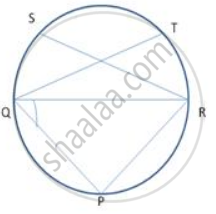Exercise 17.2 | Q 23

In following figure.,ABCD is a cyclic quadrilateral . If ∠ BCD = 100° and ∠ ABD = 70° , find ∠ ADB.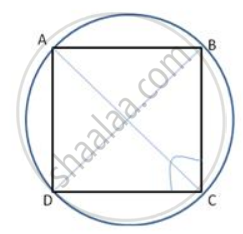Exercise 17.2 | Q 24

In following fig., O is the centre of the circle. Find ∠ CBD.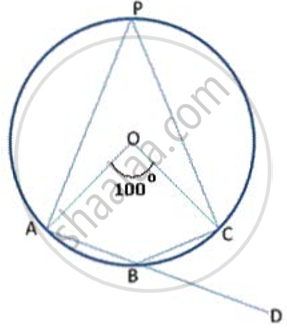Exercise 17.3

### Frank solutions for ICSE Class 10 Mathematics Part 2 Chapter 17 Circles Exercise 17.3

Exercise 17.3 | Q 1

Find the length of the tangent from a point which is at a distance of 5cm from the centre of the circle of radius 3cm.

Exercise 17.3 | Q 2

From a point P outside a circle, with centre O. tangents PA and PB are drawn as following fig., Prove that ∠ AOP = ∠ BOP and OP is the perpendicular bisector of AB.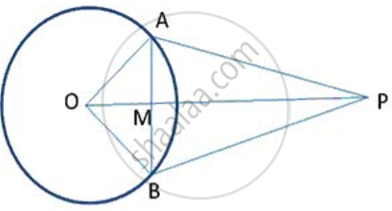Exercise 17.3 | Q 3

In fig., chords AB and CD of a circle intersect at P. AP = 5cm, BP= 3cm and CP = 2.5cm. Determine the length of DP.

Exercise 17.3 | Q 4

In following fig., chords PQ and RS of a circle intersect at T. If RS = 18cm, ST = 6cm and PT = 18cm, find the length of TQ.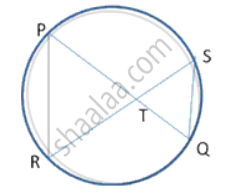Exercise 17.3 | Q 5

In fig., AB and DC are two chords of a circle with centre O. these chords when produced meet at P. if PB = Bern, BA = 7cm and PO = 14.5cm, find the radius of the circle.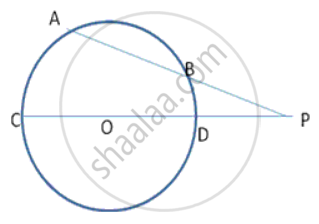Exercise 17.3 | Q 6

In following fig., PT is a tangent to the circle at T and PAB is a secant to the same circle. If PA = 4cm and AB = Scm, find PT.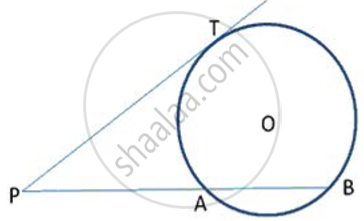Exercise 17.3 | Q 7

In following fig., PT is a tangent to the circle at T and PAB is a secant to the same circle. If PA = 4cm and AB = Scm, find PT.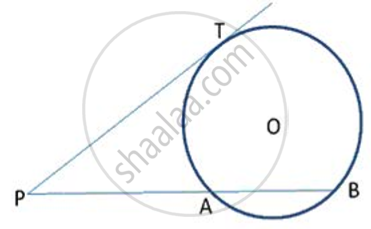Exercise 17.3 | Q 8

In following fig., PT is a tangent to the circle at T and PAB is a secant to the same circle. If PB = 9cm and AB = Scm, find PT.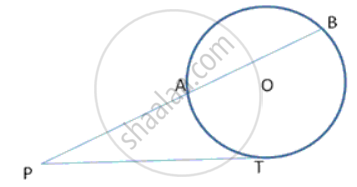Exercise 17.3 | Q 9

In following fig., PT is tangent to the circle at T and CD is a diameter of the same circle. If PC= 3cm and PT= 6cm, find the radius of the circle.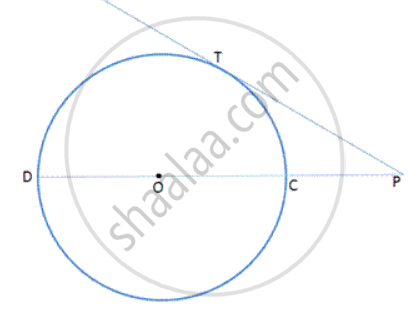Exercise 17.3 | Q 10

The length of the direct common tangent to two circles of radii 12cm and 4cm is 15cm. calculate the distance between their centres.

Exercise 17.3 | Q 11

Calculate the length of direct common tangent to two circles of radii 3cm and Bern with their centres 13cm apart.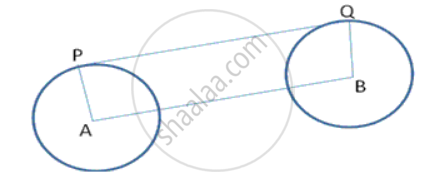Exercise 17.3 | Q 12

A point A is 17cm from the centre of the circle. The length of the tangent drawn from A to the circle is 15cm. find the radius of the circle.

Exercise 17.3 | Q 13

In the following  fig. , AC is a transversal common tangent to tvvo circles with centres P and Q and of radii 6cm and 3cm respectively. Given that AB = 8cm, calculate PQ.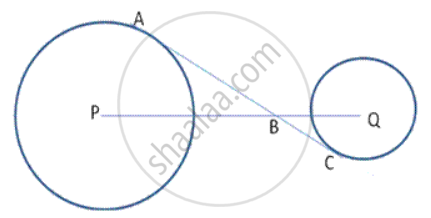Exercise 17.3 | Q 14

In following fig., ABC is a right- angled triangle at A with sides AB = 5 cm and BC = 13 cm . A circle with centre O has been inscribed in the triangle ABC. Calculate the radius of the incircle.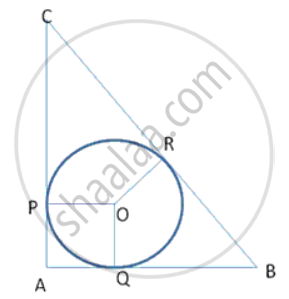Exercise 17.3 | Q 15

In followinf fig., two concentric circles with centre 0 are of radii 5 cm and 3 cm. from an external point P, tangents PA and PB are drawn to these circles. If AP = 12cm, find BP.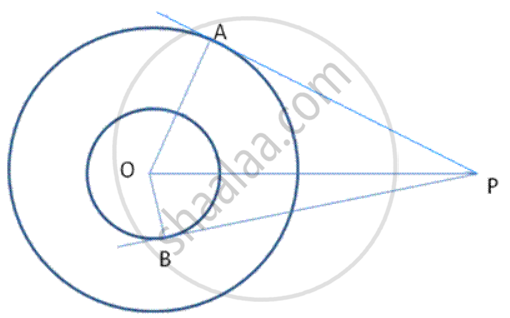Exercise 17.3 | Q 16

In the figure, XP and XQ are tangents from X to the circle with centre O. R is a point on the circle. Prove that XA + AR = XB + BR.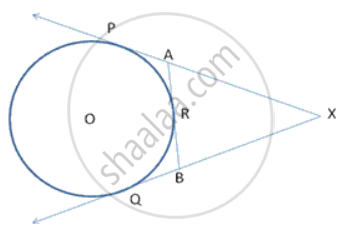Exercise 17.3 | Q 17

PA and PB are tangents from P to the circle with centre O. At M, a tangent is drawn cutting PA at K and PB at N. Prove that KN = AK + BN.

Exercise 17.3 | Q 18

Two tangents are drawn to a circle from an external point P. touching the circle at the points A and B. A third tangent intersects segment PA in C and segment PB in D and touches the circle at Q. if PA = 20 units, find the perimeter of Δ  PCD.

Exercise 17.3 | Q 19

In following figure , the incircle of Δ ABC , touches the sides BC , CA and AB at D , E and F respectively. Show AF + BD + CE = AE + BF + CD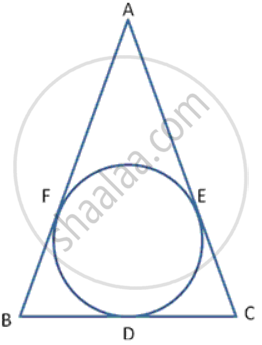Exercise 17.3 | Q 20

In following fig., a circle is touching the side BC of Δ ABC at P and AB and AC produced at Q and R respectively. Prove that AQ is half the perimeter of Δ ABC.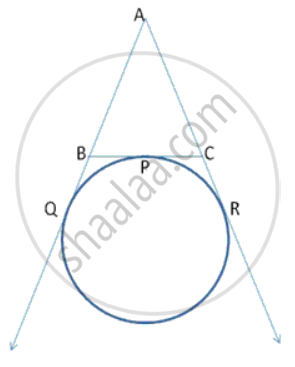Exercise 17.3 | Q 21

If all the sides of a parallelogram touch a circle, show that the parallelogram is a rhombus.

Exercise 17.3 | Q 22

If Δ PQR is isosceles with PQ = PR and a circle with centre O and radius r is the incircle of the  Δ PQR touching QR at T, prove that the point T bisects QR.

## Chapter 17: Circles

Exercise 17.1Exercise 17.2Exercise 17.3## Frank solutions for ICSE Class 10 Mathematics Part 2 chapter 17 - Circles

Frank solutions for ICSE Class 10 Mathematics Part 2 chapter 17 (Circles) include all questions with solution and detail explanation. This will clear students doubts about any question and improve application skills while preparing for board exams. The detailed, step-by-step solutions will help you understand the concepts better and clear your confusions, if any. Shaalaa.com has the CISCE ICSE Class 10 Mathematics Part 2 solutions in a manner that help students grasp basic concepts better and faster.

Further, we at Shaalaa.com provide such solutions so that students can prepare for written exams. Frank textbook solutions can be a core help for self-study and acts as a perfect self-help guidance for students.

Concepts covered in ICSE Class 10 Mathematics Part 2 chapter 17 Circles are Tangent to a Circle, Number of Tangents from a Point on a Circle, Chord Properties - a Straight Line Drawn from the Center of a Circle to Bisect a Chord Which is Not a Diameter is at Right Angles to the Chord, Chord Properties - the Perpendicular to a Chord from the Center Bisects the Chord (Without Proof), Theorem: Equal chords of a circle are equidistant from the centre., Converse: The chords of a circle which are equidistant from the centre are equal., Chord Properties - There is One and Only One Circle that Passes Through Three Given Points Not in a Straight Line, Arc and Chord Properties - the Angle that an Arc of a Circle Subtends at the Center is Double that Which It Subtends at Any Point on the Remaining Part of the Circle, Theorem: Angles in the Same Segment of a Circle Are Equal., Arc and Chord Properties - Angle in a Semi-circle is a Right Angle, Arc and Chord Properties - If Two Arcs Subtend Equal Angles at the Center, They Are Equal, and Its Converse, Arc and Chord Properties - If Two Chords Are Equal, They Cut off Equal Arcs, and Its Converse (Without Proof), Arc and Chord Properties - If Two Chords Intersect Internally Or Externally Then the Product of the Lengths of the Segments Are Equal, Cyclic Properties, Concept of Circle - Centre, Radius, Diameter, Arc, Sector, Chord, Segment, Semicircle, Circumference, Interior and Exterior, Concentric Circles, Areas of Sector and Segment of a Circle, Tangent Properties - If a Line Touches a Circle and from the Point of Contact, a Chord is Drawn, the Angles Between the Tangent and the Chord Are Respectively Equal to the Angles in the Corresponding Alternate Segments, Tangent Properties - If a Chord and a Tangent Intersect Externally, Then the Product of the Lengths of Segments of the Chord is Equal to the Square of the Length of the Tangent from the Point of Contact to the Point of Intersection, Tangent Properties - If Two Circles Touch, the Point of Contact Lies on the Straight Line Joining Their Centers.

Using Frank Class 10 solutions Circles exercise by students are an easy way to prepare for the exams, as they involve solutions arranged chapter-wise also page wise. The questions involved in Frank Solutions are important questions that can be asked in the final exam. Maximum students of CISCE Class 10 prefer Frank Textbook Solutions to score more in exam.

Get the free view of chapter 17 Circles Class 10 extra questions for ICSE Class 10 Mathematics Part 2 and can use Shaalaa.com to keep it handy for your exam preparation